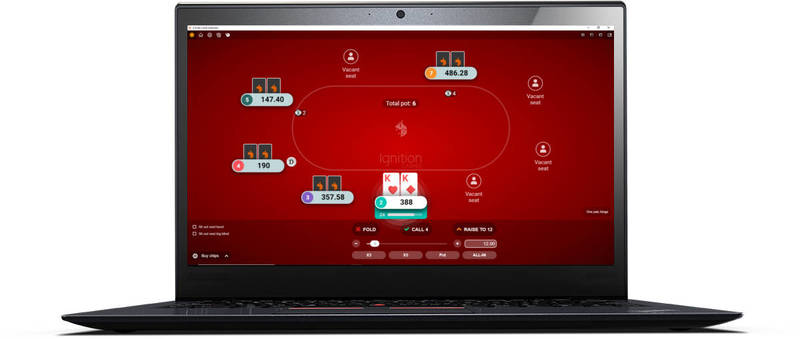# Implied Odds

I like to think that I explain implied odds a little simpler in the guide section. Try basic implied odds.Implied odds are an extension of pot odds that help you decide whether or not a drawing hand is worth calling in the face of a raise. Essentially, the implied odds of a hand tell you how much you expect to win after you make your draw.

• If you expect to win a lot more money from your opponent after you make your draw, you have good implied odds.
• If you anticipate that you will not be able to get any more money out of your opponent on future rounds, then you have little or no implied odds.

Implied odds indicate the amount of money that you expect to win after completing your draw.

## Differences between implied odds and pot odds.

Unlike in pot odds, there are no simple mathematical rules or formulas to determine what your implied odds are in Texas Hold'em (although you can work out how much you need to win from the rest of the hand to make calling profitable, which is shown later in this article).

With implied odds you pretty much make an estimate of implied odds through an understanding of your opponent and the situation. For this reason it is easier to get an idea of your implied odds during a hand if you have been playing the game for some time.

The more you play, the more you will learn about implied odds in drawing hands and eventually it will be easier to make an accurate estimation of your "implied odds".

However, if you are quite new to the game and this is the first time you have come across implied odds, here are two situations below that will help to illustrate:

1. The times when you have good implied odds
2. The times when you have little or no implied odds.

## Good implied odds situation.

You: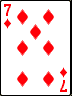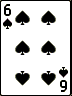Flop: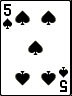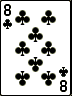In this hand you have an open ended straight draw. If your opponent bets into you, then you have good implied odds because if you make your straight, it is likely that you will be able to extract more money from your opponent on later rounds of betting. This is because your opponent will not easily be able to estimate the strength of your hand.

## Poor implied odds situation.

You: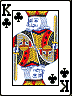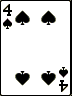Flop: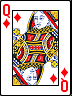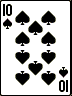Once again you have an open ended straight draw. However your implied odds are far worse in this situation because if you do make your straight when the Ace or 9 comes, the board will be very scary for your opponent as the board could easily (and obviously) make somebody the straight. There is little chance that you will get much more money out of your opponents unless they have the straight also.

In general, the more disguised your hand is, the better your implied odds are.

## How do implied odds affect your game?

The great thing about implied odds is that they have a knock on effect against your pot odds. If you anticipate you will win more money from your opponent on later rounds of betting, you can afford to make calls when your opponent is not giving you the correct pot odds to call.

For example, if you have the nut straight draw the odds that you will complete the draw on the next card are roughly 5 to 1. If your opponent bets \$25 making the pot \$100, they are giving you 4 to 1 odds to call. Now if we to base our decision purely on pot odds then we should not make the call. However, if we believe that we have good implied odds, the call becomes justifiable. This is because we will be making more money when we make our draw, than if we folded.

The fundamental rules of implied odds to remember are:

• If you have good implied odds, you can afford to call without correct pot odds.
• If you have little or no implied odds, you should stick to the pot odds.

## Calculating implied odds.

Even though it is not possible to calculate how much you are going to win with your implied odds, it is possible to calculate how much you need to win to make calling profitable. This calculation is also very straight forward.

Subtract your pot odds from the odds of hitting your draw to work out your required implied odds.

This will then give us a new ratio that we can compare with the amount we have to call to figure out how much money we need to take from our opponent later on in the hand to make the call profitable (or break even).

### Implied odds calculation example.

Let's say that we have a flush draw and our opponent bets \$10 in to a \$10 pot, which means we have to call \$10 to win a \$20 pot.

• Odds of completing our draw: 4.2:1 (see the ratio odds chart).
• Pot odds: 2:1.
• Draw odds - pot odds = 2.2:1.

So our required implied odds ratio is 2.2:1. If we multiply this 2.2 figure by the \$10 bet we have to call we get \$22.

Therefore, to make calling the \$10 bet with our flush draw a break-even play at worst, we need to extract \$22 from our opponent during the remainder of the hand.

## Conclusion.

Implied odds are useful for deciding whether to call a bet after you have calculated the pot odds. If you do not have the correct odds to call a bet and make your draw, then a call is justifiable if you have good implied odds. However you should stick to the pot odds if you anticipate that you have little or no implied odds.

It should be noted that you have no implied odds if your opponent is all-in, because there will be no betting on further rounds if nobody else in the pot. Be sure to be careful when calling large raises, as the implied odds that you are getting may not cover the amount you have to call in order to make your draw. The best way to obtain a greater understanding of your pot odds and implied odds is to simply play more poker.

For the next article on everything odds in poker, have a read over reverse implied odds.

Go back to the awesome Texas Hold'em Strategy.